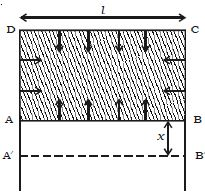# Relation between Surface Energy and Surface Tension

The potential energy per unit area of the surface film is called surface energy. Consider a metal frame ABCD in which AB is movable. The frame is dipped in a soap solution. A film is formed which pulls AB inwards due to surface tension. If T is the surface tension of the film and l is the length of the wire AB. this inward force is given by 2 x Tl. The number 2 indicates the two free surfaces of the film.Fig: Surface energy

If AB is moved through a small distance x as shown in Figure, to the position AB’, then work done is, W = 2Tlx

Work done per unit area: W/Tlx

So, Surface energy = T2lx/2lx

Surface energy = T

Surface energy is numerically equal to surface tension.